Author: Oscar Cronquist Article last updated on April 08, 2021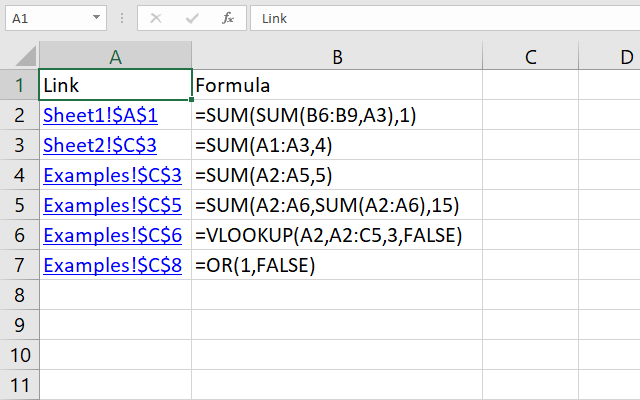This article describes different ways to locate literal or hardcoded values in formulas. The image above shows the result from a macro that iterates through each worksheet in a given workbook looking for hardcoded values in formulas.

A link is generated and the formula itself is displayed if a hardcoded value is found.

## 1. Find hardcoded values in formulas (Conditional Formatting)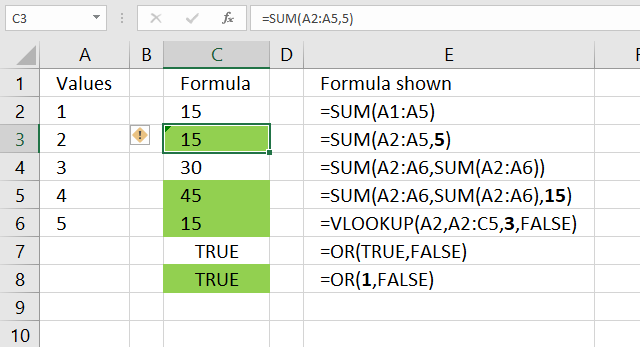I found this UDF in David Hager's Excel Experts E-letter (EEE) on J Walkenbach's website. You need to apply conditional formatting with a formula that uses the User Defined Function described below.

Conditional Formatting highlights cells with formulas containing hardcoded values, the image above shows which cells contain hardcoded values. They are highlighted green. For example, cell C3 contains the following formula:

=SUM(A2:A5, 5)

The second argument in the SUM function is a hardcoded value, cell C3 is highlighted.

Note, Conditional Formatting is super-volatile and may slow down your workbook considerably. It only highlights cells, you need to find the highlighted cells yourself.

I recommend the macro in section 2. The macro creates a new worksheet and lists all formulas containing hardcoded values as well as links to those cells.

```'Name User Defined Function
Function CellUsesLiteralValue(Cell As Range) As Boolean

'Check if cell has not a formula
If Not Cell.HasFormula Then

'Save boolean value FALSE to variable CellUsesLiteralValue
CellUsesLiteralValue = False

'Continue here if cell has a formula
Else

'Use like operator to determine if cell formula contains hardcoded values, it returns TRUE if found.
'Characters enclosed in brackets allows you to match any single character in the string.
' The hashtag matches any single digit, the asterisk matches zero or more characters
CellUsesLiteralValue = Cell.Formula Like "*[=^/*+-/()<>, ]#*"
End If
End Function
```

Where to put the VBA code?

Back to top

### 1.1 How can I locate cells containing formulas with literal values?

Use the UDF as your conditional formatting formula, I will explain exactly how in the next section below if you are interested. It accepts a single cell as an argument. It returns True if the cell's formula contains an operator followed by a numerical digit. In other words, it identifies cells that have a formula that contains a literal numeric value.

You can test each cell in the range, and highlight it if the function returns True. The UDF with conditional formatting highlights cells containing formulas with literals, column C. You can download an excel file at the end of this post if you are interested in this technique.

I have bolded all literal values in the formulas, column E. It seems to work with nested formulas also. Boolean values can be expressed as TRUE/FALSE but also 1/0 in Excel. Note, some formulas are built to have hard-coded arguments, like col_index_num in the VLOOKUP function.

Back to top

### 1.2 How to use the User Defined Function with Conditional Formatting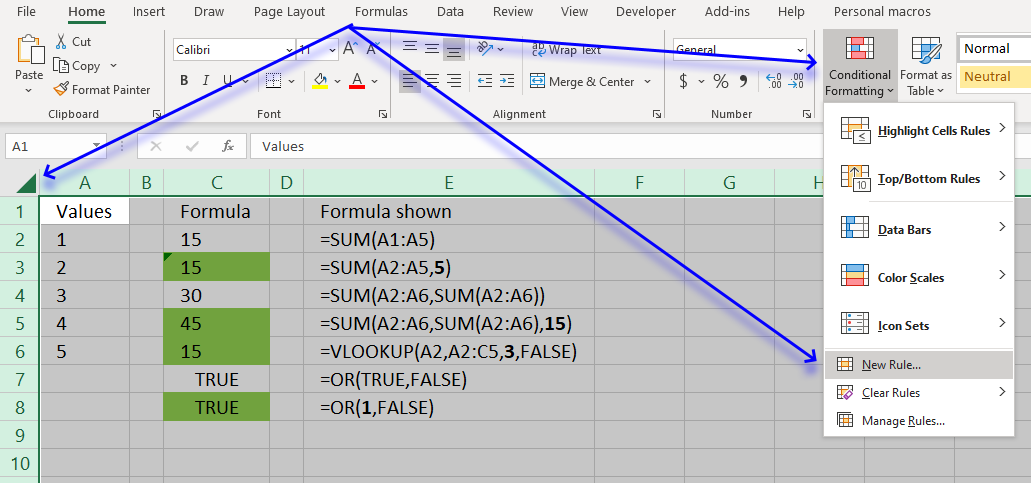1. Go to the worksheet you want to search.
2. Click the button containing a triangle to select all cells on the worksheet, see image above.
3. Go to tab "Home" on the ribbon if you are not already there.
4. Click "Conditional Formatting" button, see image above. A pop-up menu appears.
5. Click "New Rule...", see image above. A dialog box shows up on the screen.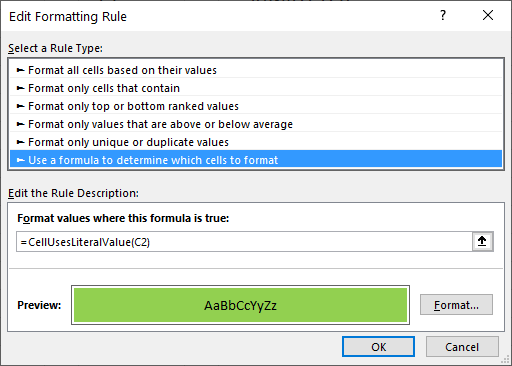The image above shows the wrong cell reference C2, it should be A1.
6. Click "Use a formula to determine which cells to format".
7. Type following formula: =CellUsesLiteralValue(A1)
8. Click "Format..." button. Another dialog box appears.
9. Go to tab "Fill".
10. Pick a color.
11. Click "OK" button.
12. Click "OK" button again.

Back to top

## 2. Find hardcoded values in formulas across worksheetsI have a large workbook and I am in a hurry!

The authors want you to use it as conditional formatting formula to spot cells containing formulas with literal values, that can be tedious work with a large workbook. I would like to show you how to use the udf to build a list of all cells containing literal values, from an entire workbook.

This picture above shows you what my macro below returns, first a new sheet is inserted. It is then populated with links to all cells containing formulas with literals and their corresponding formulas.

I have reused some parts of Rick Rothstein's macro.

```'Name macro
Sub FindLiteralsInWorkbook()

'Dimension variables and declare data types
Dim C As Range, A As Range, Addresses As String, i As Single
Dim cell As Range

'Add worksheet to workbook
Sheets.Add

'The SET statement allows you to save an object reference to a variable, in this case the active worksheet
Set x = ActiveSheet

'Save text "Link" to cell A1.
x.Range("A1") = "Link"

'Save text "Formula" to cell B1.
x.Range("B1") = "Formula"

'Save number 1 to variable i.
i = 1

'Iterate through worksheets in active workbook
For Each sh In ActiveWorkbook.Worksheets

'Enable error handling
On Error Resume Next

'Find cells containing constants and save to object C
Set C = sh.Cells.SpecialCells(xlConstants)

'Check if C is empty
If C Is Nothing Then

'Find cells containing formulas and save to object C
Set C = sh.Cells.SpecialCells(xlFormulas)

'Continue here if C is not empty
Else

'Returns the union of the two cell ranges and saves to object C
Set C = Union(C, sh.Cells.SpecialCells(xlFormulas))
End If

'Iterate through all the ranges in a multiple-area selection.
For Each A In C.Areas

'Iterate through all cells in cell range A
For Each cell In A

'Use User Defined Function to determine if cell contains at least one hardcoded value
If CellUsesLiteralValue(cell) = True Then

'Add 1 to variable i
i = i + 1

'Create a hyperlink based on the cell address
x.Hyperlinks.Add Anchor:=x.Range("A" & i), _
Address:=ActiveWorkbook.Path & "\" & ActiveWorkbook.Name, _
SubAddress:=sh.Name & "!" & cell.Address, _
TextToDisplay:=sh.Name & "!" & cell.Address
x.Range("B" & i) = "'" & cell.Formula
End If

'Continue with next cell
Next cell

'Continue with next cell range
Next A

'Disable error handling
On Error GoTo 0

'Continue with next worksheet
Next sh

'Resize column A:E widths
x.Columns("A:E").AutoFit
End Sub
```

Back to top

## 3. Where to put the code?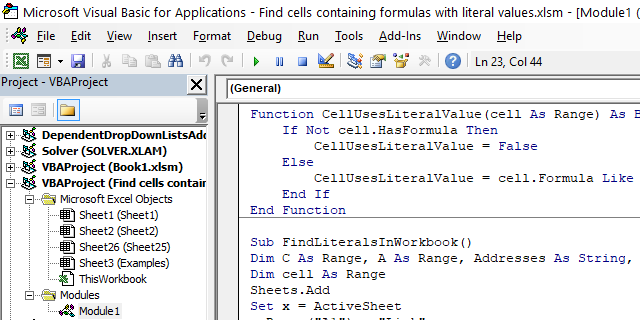1. Copy User Defined Function CellUsesLiteralValue.
2. Go to the VB Editor (Alt + F11).
3. Go to the menu and click Insert.
4. Click Module.
5. Paste UDF to your workbook's code module
6. Copy macro FindLiteralsInWorkbook
7. Paste to the module. Both the UDF and macro should now be visible in the module, see image above.
8. Return to Excel.

Back to top

## 4. How to run the macro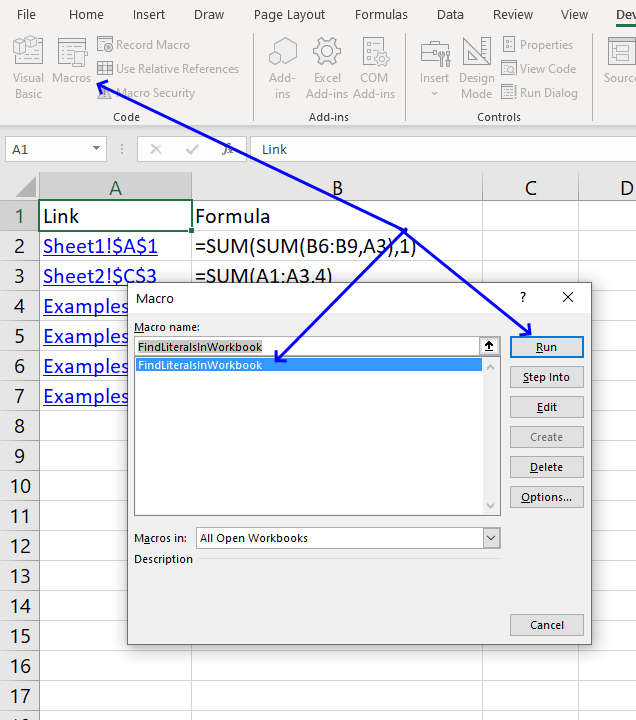1. Go to tab Developer on the ribbon.
2. Click "Macros" button.
3. Click "FindLiteralsInWorkbook" and then click "Run".

Back to top

### Download Excel fileFind-cells-containing-formulas-with-literal-valuesv2.xlsm

### Recommended reading

Hyperlinks.Add Method (Excel)

Back to top